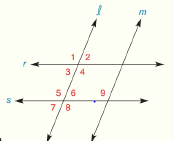Chapter 2.CT, Problem 2CT### Elementary Geometry for College St...

6th Edition
Daniel C. Alexander + 1 other
ISBN: 9781285195698

#### Solutions

Chapter
Section### Elementary Geometry for College St...

6th Edition
Daniel C. Alexander + 1 other
ISBN: 9781285195698
Textbook Problem
1 views

# In the accompanying figure, m ∠ 2 = 68 ° , m ∠ 8 = 112 ° , and m ∠ 9 = 110 ° .a).Which lines (r and s OR l and m) must be parallel? ___________b) Which pair of lines (r and s OR l and m) cannot be parallel? ___________To determine

a)

To find:

The parallel lines.

Explanation

Given:

The following figure shows the given diagram.

m2=68°m8=112°m9=110°

Approach:

The lines s and r are cut by the transversal line l, and according to the parallel line theorem, the lines s and r will be supplementary if exterior angles on the same side of the l are supplementary. The angles 2 and 8 are outside the line then these angles are exterior angles. The angles 2 and 8 are on the same side of the l, that means exterior angles are on same side of the transversal

To determine

b)

To find:

The lines that are not parallel.

### Still sussing out bartleby?

Check out a sample textbook solution.

See a sample solution

#### The Solution to Your Study Problems

Bartleby provides explanations to thousands of textbook problems written by our experts, many with advanced degrees!

Get Started

#### Evaluate the indefinite integral. a+bx23ax+bx3dx

Single Variable Calculus: Early Transcendentals, Volume I

#### Find the matrix A if A=

Finite Mathematics for the Managerial, Life, and Social Sciences

#### True or False:

Study Guide for Stewart's Multivariable Calculus, 8th